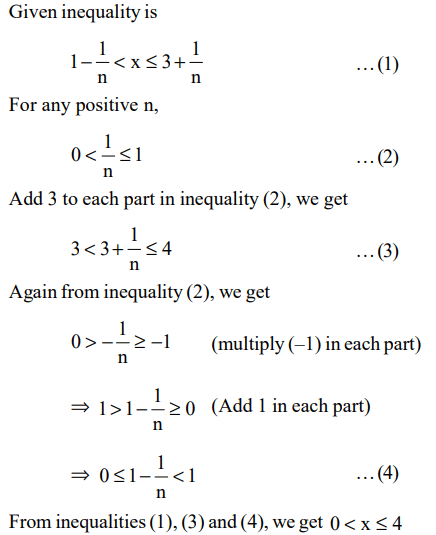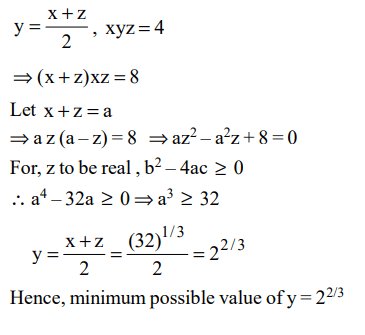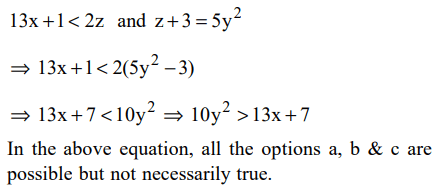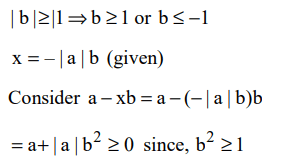## Algebra Questions and Answers Part-5

1. The number of roots common between the two equations $x^{3}+3x^{2}+4x+5=0$     and $x^{3}+2x^{2}+7x+3=0$     is
a) 0
b) 1
c) 2
d) 3

Explanation: Subtract the both equations, we get
x2-3x+2 = 0
(x - 1)(x - 2) = 0
x = 1, 2
Roots 1 and 2 do not satisfy any of the original equation.
In case there is a common root, it will be the root of the subtracted equation.
Therefore there is no common root between both the given equations.

2. A real number x satisfying $1-\frac{1}{n}$ < $x\leq 3+\frac{1}{n}$   , for every positive integer n, is best described by
a) 1 < x < 4
b) 1 < $x\leq3$
c) 0 < $x\leq4$
d) $1\leq x\leq3$

Explanation:3. If x and y are integers then the equation 5x + 19y = 64 has
a) no solution for x < 300 and y < 0
b) no solution for x > 250 and y > – 100
c) a solution for 250 < x < 300
d) a solution for – 59 < y < – 56

Explanation: 5x + 19y = 64
We see that if y = 1, we get an integer solution x = 9, Now if y changes (increases or decreases) by 5, x will change (decrease or increase) by 19
Looking at options, if x = 256, we get, y = 64
Using these values we see option a, b and d which are eliminated and also that there exists a solution for
250 < x < 300.

4. If both a and b belong to the set {1, 2, 3, 4}, then the number of equations of the form $a x^{2}+b x+1=0$    having real roots is
a) 10
b) 7
c) 6
d) 12

Explanation:5. If three positive real numbers x, y, z satisfy y – x = z – y and x y z = 4, then what is the minimum possible value of y?
a) $2^{1/3}$
b) $2^{2/3}$
c) $2^{1/4}$
d) $2^{3/4}$

Explanation:6. If n is such that $36\leq n\leq72$   , then $x=\frac{n^{2}+2\sqrt{n}\left(n+4\right)+16}{n+4\sqrt{n+4}}$     satisfies
a) 20 < x < 54
b) 23 < x < 58
c) 25 < x < 64
d) 28 < x < 60

Explanation:7. If 13x + 1 < 2z and $z+3=5y^{2}$ , then
a) x is necessarily less than y
b) x is necessarily greater than y
c) x is necessarily equal to y
d) None of the above is necessarily true

Explanation:8. If $\mid b\mid\geq 1$  and $x=-\mid a \mid b$    , then which one of the following is necessarily true?
a) a – xb < 0
b) $a-xb\geq 0$
c) a – xb > 0
d) $a-xb\leq 0$

Explanation:9. The total number of integer pairs (x, y) satisfying the equation x + y = xy is
a) 0
b) 1
c) 2
d) None of the above

Explanation: Equation x + y = xy can be satisfied for (x, y) $\equiv$ (0, 0), (2, 2)
10. If $\frac{a}{b+c}=\frac{b}{c+a}=\frac{c}{a+b}=r$     , then r cannot take any value except
a) $\frac{1}{2}$
c) $\frac{1}{2} or -1$
d) $-\frac{1}{2} or -1$